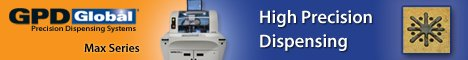Printed Circuit Board Assembly & PCB Design Forum

SMT electronics assembly manufacturing forum.

# Aspect Ratio Calculation

Views: 2452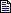I read about 10:1 , 6:1 aspect ratio but I don't understand ... - May 28, 2006 by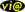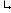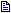Aspect ratio: The ratio of a via�s length or depth to its pr... - May 30, 2006

Sjm

Aspect Ratio Calculation | 28 May, 2006

I read about 10:1 , 6:1 aspect ratio but I don't understand how it works. Can someone explain how you figure out the aspect ratio on a design with a board thickness of .093 and via hole size of .010 I don't know how to get the 6:1 number

Is it the board thickness devided by smallest hole size? If so how does 6:1 or 10:1 get figured in

Thanks

This message was postedthe Electronics Forum @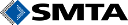Aspect Ratio Calculation | 30 May, 2006

Aspect ratio: The ratio of a via�s length or depth to its pre-plated diameter.

So, in your case, either * 6=0.093/x, calculating x=0.093/6=0.016 as the smallest via hole diameter. ... OR * 6=y/0.01, calculating y=6*0.01=0.06 as the maximum board thickness.